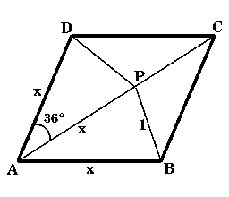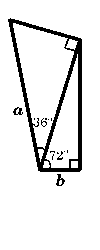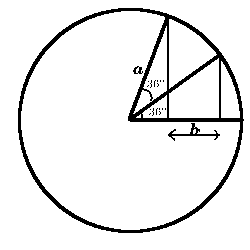You may also likeDegree Ceremony

What does Pythagoras' Theorem tell you about these angles: 90°, (45+x)° and (45-x)° in a triangle?Logosquares

Ten squares form regular rings either with adjacent or opposite vertices touching. Calculate the inner and outer radii of the rings that surround the squares.Ball Bearings

If a is the radius of the axle, b the radius of each ball-bearing, and c the radius of the hub, why does the number of ball bearings n determine the ratio c/a? Find a formula for c/a in terms of n.

Gold Again

Age 16 to 18 Challenge Level:Consider the rhombus as illustrated where $x$ is an unknown length, $AP = AD = x$ , angle $DAP = 36$ degrees and $P$ is a point on the diagonal $AC$ such that $PB = 1$ unit. Without using a calculator, computer or tables find the exact values of 1. $\cos36^{\circ}\cos72^{\circ}$ 2. $\cos36^{\circ} - \cos72^{\circ}.$3. Draw these two diagrams as accurately as you can and measure the lengths $a$ and $b$. What do you notice? Can you prove it? (In each diagram there are two right angled triangles).